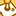QlikView App Dev

Discussion Board for collaboration related to QlikView App Development.

Announcements
QlikWorld 2022, LIVE in Denver CO., May 16-19, 2022. REGISTER NOW TO RECEIVE EARLY BIRD PRICING
cancel
Showing results for
Did you mean:Creator

Matrix Show all Values(x,y axes) but hide null

Dear Community,

i need to build a matrix with A and B axes.

All the time all possible values of A and B should be visible, so the matrix should be fully expanded.

For such a model i use a pivot with two dimensions A&B and an expression with simple 'x'.

The dimensions A and B have simple inline data 1,2,3,4,5 and i checked "show all values" for them.

Also there is data for A and B combinations but not for all possible combinations.=> The Problem is that also null values of A & B are displayed

If i check "Suppress When Value is Null" for the dimensions, i lose the benefit of "show all values"  as for these dimensions

combination where we dont have data, the dimension value is not displayed.so how can i make it pssible to have all possible dimensions without NULL inside the matrix

with data that is not for all combinations of A&B present.

Best regards!

1 Solution

Accepted SolutionsMVP

Try this out:

=If(Len(Trim(B)) > 0, If(COUNT(X) = 0, '-', Count(X)))11 RepliesMVP

Would you be able to share the sample you are working with above?Creator
Author

Hi Sunny, i have added a sample script.

I appreciate your helpMVP

is thisCreator
Author

Hi Chanty, this is not correct as the Dimension A loses the value 5!MVP

Try the attachedCreator
Author

Hi Sunny,

'x' was just an example try now the values X

So using your expression =If(Len(Trim(B)) > 0, X)  you will see it's not working as the matrix does not show all dimensions as there are not for all combination values available.MVP

Try this expression in that case:

=If(Len(Trim(B)) > 0, If(Len(Trim(X)) > 0, X, ' '))Creator
Author

Thanks Sunny,

this goes into the right direction but still nneds some improvement:

There are some values missing inside the matrix for example there are multiple values for A=2 & B=2

So we use a count(X) but then we still need to hide the columns where the dimension is null

Check the file & thanks for your support!MVP

Try this out:

=If(Len(Trim(B)) > 0, If(COUNT(X) = 0, '-', Count(X)))Community Browser